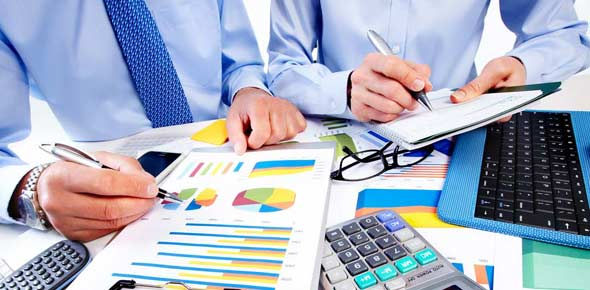# MGT101- Financial Accounting 2

22 Questions | Total Attempts: 1083SettingsThis is a interesting and important quiz for accounting. Take this quiz and have a good time!

• 1.
A transaction that has been recorded in wrong account of the same class instead of being recorded in the right account
• A.

Error of Omission

• B.

Error of Commission

• C.

Error of Principle

• D.

Error of Original entry

• 2.
Which of the following is a debit entry on the Bank Statement that might not appear in the Cash Book?
• A.

A standing order

• B.

An uncredited cheque

• C.

A credit transfer

• D.

An unpresented cheque

• 3.
Which of the following account balance is shown on debit side of Trial Balance?(It is assumed that all account balances are shown on normal balance).
• A.

Capital account

• B.

Sundry creditors account

• C.

Accounts payable account

• D.

Sundry debtors account

• 4.
If cost of sales is Rs. 95,000, income from sales Rs. 200,000 and operatingexpenses Rs. 300,000. What will be net result?
• A.

Rs.195,000 Losses

• B.

Rs.195,000 Profits

• C.

Rs.105,000 Profits

• D.

Rs.105,000 Losses

• 5.
In which of the following statement opening stock is shown?
• A.

Profit and loss account

• B.

Balance sheet

• C.

Cash flow statement

• D.

Owner’s equity

• 6.
Working capital Rs. 20,000, Current liabilities Rs. 30,000 and fixed assets Rs.100,000; calculate current assets?
• A.

Rs. 10,000

• B.

Rs. 50,000

• C.

Rs. 110,000

• D.

Rs. 120,000

• 7.
Which of the following content(s) is (are) included in the Cost of goods sold?
• A.

Opening stock

• B.

Purchases

• C.

Freight in

• D.

All of the given options

• 8.
Which of the following Organization converts raw material into finished goods?
• A.

• B.

Manufacturing concern

• C.

Merchandising concern

• D.

Service concern

• 9.
Which of the following is an example of direct materials cost?
• A.

Production worker’s wages

• B.

Depreciation expenses

• C.

A piece of wood for the production of chair

• D.

Polish and finishing material for chair

• 10.
In cost of goods sold statement, the ‘cost of material consumed’ is equal to:
• A.

Opening raw material inventory + Purchases – Ending raw material inventory

• B.

Opening raw material inventory - Purchases + Ending raw material inventory

• C.

Ending raw material inventory - Opening raw material inventory – Purchases

• D.

Ending raw material inventory + Opening raw material inventory + Purchases

• 11.
What would be the value of Total Factory Cost, if cost of raw materials, directlabor costs, and manufacturing overhead costs are Rs.80,000, Rs.50,000, andRs.60,000 respectively?
• A.

Rs.130, 000

• B.

Rs.110, 000

• C.

Rs.140, 000

• D.

Rs.190, 000

• 12.
Which of the following assets are shown at written down value in Balance Sheet?
• A.

Current assets

• B.

Liquid assets

• C.

Floating assets

• D.

Fixed assets

• 13.
An asset cost Rs. 50,000, has an estimated residual value of Rs.1, 500, and anestimated useful life of 5 years. What is the depreciation rate?
• A.

20.0%

• B.

25.0%

• C.

35.5%

• D.

50.4 %

• 14.
Calculate depreciation of machine after first year by using diminishing balancemethod with the help of given data?If, Cost of machine = Rs.400, 000Useful life = 5 yearsResidual value = Rs.25, 000Sale price = Rs.40, 000Rate of depreciation = 40%
• A.

Rs. 160,000

• B.

Rs. 11,840

• C.

Rs. 34,560

• D.

Rs. 34,860

• 15.
Which one of the following is INCORRECT about closing Stock?
• A.

It is added into current assets

• B.

It is deducted from material available for use

• C.

It becomes opening stock of next year

• D.

It increases the owner’s equity of business

• 16.
In the cost of goods sold statement, Cost of direct material consumed + Directlabor = ___________
• A.

Conversion cost

• B.

Prime cost

• C.

Total factory cost

• D.

Cost of goods manufactured

• 17.
The total of all costs incurred to convert raw material into finished goods isknown as:
• A.

Prime cost

• B.

Conversion cost

• C.

Sunk cost

• D.

Opportunity cost

• 18.
In cost of goods sold statement the ‘total factory cost’ is equal to:
• A.

Cost of material consumed + Labor cost

• B.

Cost of material consumed + Conversion cost

• C.

Cost of material consumed + Total factory cost

• D.

Cost of material consumed + Factory overhead

• 19.
Which of the following is (are) inventory valuation method(s)?
• A.

FIFO

• B.

LIFO

• C.

Weighted average

• D.

All of the given options

• 20.
The cost of an incomplete fixed asset is transferred to _________ as on BalanceSheet date.
• A.

Capital account

• B.

Capital work in progress account

• C.

Relevant asset account

• D.

Owner's equity account

• 21.
Under the straight line method of depreciation:
• A.

Amount of depreciation increases every year

• B.

Amount of depreciation remains constant for every year

• C.

Amount of depreciation decreases every year

• D.

None of the given options

• 22.
Which of the following asset is NOT depreciated?
• A.

Factory Buildings

• B.

Office Equipment

• C.

Land

• D.

Plant & Machinery

Related TopicsBack to top